# MCQ in Fundamentals in Algebra Part 1 | Math Board Exam

(Last Updated On: January 1, 2021)This is the Multiples Choice Questions Part 1 of the Series in Fundamentals in Algebra as one of the Engineering Mathematics topic. In Preparation for the ECE Board Exam make sure to expose yourself and familiarize in each and every questions compiled here taken from various sources including but not limited to past Board Examination Questions in Engineering Mathematics, Mathematics Books, Journals and other Mathematics References.

#### MCQ Topic Outline included in Mathematics Board Exam Syllabi

• MCQ in Basic Rules in Algebra | MCQ in Properties of Equality | MCQ in Properties of Zero | MCQ in Properties of Exponent | MCQ in Properties of Radicals | MCQ in Surds | MCQ in Special Products | MCQ in Properties of Proportion | MCQ in Remainder Theorem | MCQ in Factor Theorem

#### Start Practice Exam Test Questions Part 1 of the Series

Choose the letter of the best answer in each questions.

Problem 1: ECE Board April 1999

If 16 is 4 more than 4x, find 5x – 1.

A. 14

B. 3

C. 12

D. 5

Solution:Review Solution for Number 1

Problem 2: EE Board October 1992

Find the value of x in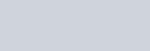A. 16.47

B. 12.87

C. 18.27

D. 20.17

Solution:Review Solution for Number 2

Problem 3: EE Board October 1991

Find the value of x in the equations: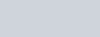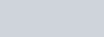A. 50/9

B. 80/9

C. 70/9

D. 60/9

Solution:Review Solution for Number 3

Problem 4: EE Board October 1997

Find the values of x and y from the equations:

x – 4y + 2 = 0

2x + y – 4 = 0

A. 11/7, -5/7

B. 14/9, 8/9

C. 4/9, 8/9

D. 3/2, 5/3

Solution:Review Solution for Number 4

Problem 5: ME Board October 1995

Solve for the value of x and y.

4x + 2y = 5 13x -3y = 2

A. y = 1/2, x = 3/2

B. y = 3/2, x = 1/2

C. y = 2, x = 1

D. y = 3, x =1

Solution:Review Solution for Number 5

Problem 6: ME Board October 1996

Solve the simultaneous equations:

2x2 – 3y2 = 6

3x2 + 2y2 = 35

A. x = 3 or -3; y = 2 or -2

B. x = 3 or -3, y = -2 or 1

C. x = 3 or -3, y = -2 or -1

D. x = 3 or -3, y = 2 or -3

Solution:Review Solution for Number 6

Problem 7: CE Board May 1997

Find the value of w in the following equations:

3x – 2y + w = 11

x + 5y – 2w = -9

2x + y -3w = -6

A. 3

B. 2

C. 4

D. -2

Solution:Review Solution for Number 7

Problem 8: EE Board October 1993

Solve for the value of x.

2x – y + z = 6

x- 3y – 2z = 13

2x – 3y – 3z = 16

A. 4

B. 3

C. 2

D. 1

Solution:Review Solution for Number 8

Problem 9: ME Board October 1996

Solve the simultaneous equations:

x + y = – 4

x + z – 1 = 0

y + z + 1 = 0

A. x = -1, y = -5, z = 3

B. x = 1, y = 2, z = -3

C. x = -1, y = -3, z = 2

D. x = -2, y = -3, z = -1

Solution:Review Solution for Number 9

Problem 10: EE Board April 1997

Multiply the following: (2x + 5y)(5x – 2y)

A. 10x2 – 21xy + 10y2

B. -10x2 + 21xy + 10y2

C. 10x2 + 21xy – 10y2

D. -10x2 – 21xy – 10y2

Solution:Review Solution for Number 10

Problem 11: EE Board March 1998

Determine the sum of the positive valued solution

to the simultaneous equations:

xy = 15, yz = 35, zx = 21.

A. 16

B. 13

C. 17

D. 19

Solution:Review Solution for Number 11

Problem 12: ECE Board April 1991

Simplify:Solution:Review Solution for Number 12

Problem 13: ECE Board November 1993

Simplify the following equation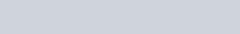Solution:Review Solution for Number 13

Problem 14: ECE Board April 1991

Solution:Review Solution for Number 14

Problem 15: ECE Board April 1991

Simplify: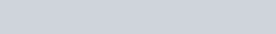A. -5a

B. -3a

C. -7a

D. -4a

Solution:Review Solution for Number 15

Problem 16:

Solve for x: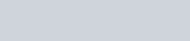A. b + 4

D. b – 4

Solution:Review Solution for Number 16

Problem 17: ECE Board April 1993

Solve for y: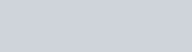A. x – z

B. x + z

C. a + b

D. a – b

Solution:Review Solution for Number 17

Problem 18: ME Board October 1996

Solution:Review Solution for Number 18

Problem 19: CE Board May 1996

Find the value of A in the equation: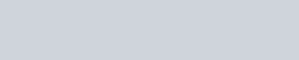A. -2

B. 1/2

C. -1/2

D. 2

Solution:Review Solution for Number 19

Problem 20: ME Board October 1996

The value of (3 to 2.5 power) square is equal to:

A. 729

B. 140

C. 243

D. 81

Solution:Review Solution for Number 20

Problem 21:

Evaluate: 64x • 4y

A. 256xy

B. 4x + 3y

C. 64x + 3y

D. 43x + y

Solution:Review Solution for Number 21

Problem 22: ECE Board April 1993

Solve for x in the following equations.

27x = 9y

81y3 – x = 243

A. 1

B. 1.5

C. 2

D. 2.5

Solution:Review Solution for Number 22

Problem 23: ECE Board April 1993

Evaluate: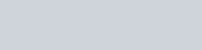A. y = 5n

B. y = 9

C. y = 52n

D. y = 18

Solution:Review Solution for Number 23

Problem 24: ECE Board April 1990

Given: (an)( am) = 100,000 anm = 1000000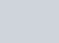Find a:

A. 12

B. 9

C. 11

D. 10

Solution:Review Solution for Number 24

Problem 25: ECE Board November 1991

Give the factors of a2 – x2

A. 2a – 2x

B. (a + x)(a – x)

C. (a – x)(a + x)

D. 2x – 2a

Solution:Review Solution for Number 25

Problem 26: ME Board October 1996

Factor the expression x2 + 6x + 8 as completely possible.

A. (x + 4)(x + 2)

B. (x – 4)(x + 2)

C. (x – 4)(x – 2)

D. (x + 6)(x + 2)

Solution:Review Solution for Number 26

Problem 27: ECE Board November 1990

Expand: (a – b)3 = ?

A. a3 – 3a2b + 3ab2 + b3

B. a3 – 3a2b – 3ab2 – b3

C. a3 + 3a2b + 3ab2 – b3

D. a3 – 3a2b + 3ab2 – b3

Solution:Review Solution for Number 27

Problem 28:

Find the value of k so that 4x2 + 6x + k is a perfect square.

A. 36

B. 2.5

C. 9

D. 2.25

Solution:Review Solution for Number 28

Problem 29: ME Board April 1995

Factor the expression 3x3 – 3x2 – 18x

A. 3x(x – 3)(x + 2)

B. 3x(x + 3)(x + 2)

C. 3x(x + 3)(x – 2)

D. 3x(x – 3)(x – 2)

Solution:Review Solution for Number 29

Problem 30:

If p – q = 5 and pq = k/2, then p2 + q2 equals,

A. k

B. 25k

C. 25 + k

D. k/25

Solution:Review Solution for Number 30

Problem 31: ME Board April 1995

Simplify: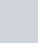Solution:Review Solution for Number 31

Problem 32: ME Board April 1998

Find the value of x which will satisfy the following expression: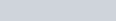A. 3/2

B. 9/4

C. 18/6

D. None of these

Solution:Review Solution for Number 32

Problem 33:

Simplify: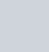Solution:Review Solution for Number 33

Problem 34: ME Board April 1996

If x to the ¾ power equals 8, x equals

A. -9

B. 6

C. 9

D. 16

Solution:Review Solution for Number 34

Problem 35:

Solve for x: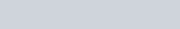A. 3

B. 23

C. 3 and 23

D. 20

Solution:Review Solution for Number 35

Problem 36: CE Board November 1991

Solve for x from the given equation:A. 4

B. 2

C. 3

D. 5

Solution:Review Solution for Number 36

Problem 37: EE Board October 1997

If f(x) = 2x2 + 2x + 4, what is f(2)?

A. 4x + 2

B. 16

C. x2 + x + 2

d. 8

Solution:Review Solution for Number 37

Problem 38: EE Board April 1997

If n is any positive integer, when

(n – 1) (n – 2) (n – 3)… (3)(2)(1) =

A. e(n – 1)

B. (n – 1)!

C. n!

D. (n – 1)n

Solution:

Problem 39:

What is the least common multiple of 15 and 18?

A. 3

B. 5

C. 90

D. 270

Solution:Review Solution for Number 39

Problem 40: ECE Board April 1998

What is the lowest common factor of 10 and 32?

A. 320

B. 2

C. 180

D. 90

Solution:Review Solution for Number 40

Problem 41:

The numbers 12 and 16 has the greatest common divisor of

A. 2

B. 4

C. 6

D. 192

Solution:Review Solution for Number 41

Problem 42: EE Board April 1996, EE Board March 1998

The Polynomial x3 + 4×2 – 3x + 8 is divided by (x – 5), then the remainder is,

A. 175

B. 140

C. 218

D. 200

Solution:Review Solution for Number 42

Problem 43:

Find the quotient of 3x5 – 4x3 + 2x2 + 36x + 48 divided by x3 – 2x2 + 6.

A. 3x2 – 4x – 8

B. 3x2 + 4x + 8

C. 3x2 – 6x – 8

D. 3x2 + 6x + 8

Solution:Review Solution for Number 43

Problem 44: CE Board November 1997

Find the remainder if we divide 4y3 + 18y2 + 8y – 4 by (2y + 3).

A. 10

B. 11

C. 15

D. 13

Solution:Review Solution for Number 44

Problem 45: ECE Board November 1999

Given: f(x) = (x + 3)(x – 4) + 4. When f(x) is divided by (x – k), the remainder is k. Find k.

A. 2

B. 4

C. 6

D. 8

Solution:Review Solution for Number 45

Problem 46:

The expression x4 + ax3 + 5x2 + bx + 6 when divided by (x – 2) leaves a remainder of 16 and when divided by (x + 1) leaves a remainder of 10. Find a and b.

A. a = 5, b = 7

B. a = -5, b = 7

C. a = -5, b = -7

D. a = 5, b = -7

Solution:Review Solution for Number 46

Problem 47:

The mean of x and y is a, the mean of y and z is b and the mean of x and z is c. What is the mean of x, y, and z?

Solution:Review Solution for Number 47

Problem 48: ECE Board April 1999

Find the mean proportional of 4 and 36.

A. 72

B. 24

C. 12

D. 20

Solution:Review Solution for Number 48

Problem 49: ECE Board April 1998

The arithmetic mean of 80 numbers is 55. If two numbers namely 250 and 850 are removed, what is the arithmetic mean of the remaining numbers?

A. 42.31

B. 50

C. 38.62

D. 57.12

Solution:Review Solution for Number 49

Problem 50: ECE Board April 1998

The arithmetic mean of 6 numbers is 17. If two numbers are added to the progression, the new set of numbers will have an arithmetic mean of 19. What are the two numbers if their difference is 4?

A. 21, 25

B. 23, 27

C. 8, 12

D. 16, 20

Solution:Review Solution for Number 50

### Online Questions and Answers in Fundamentals in Algebra Series

Following is the list of practice exam test questions in this brand new series:

MCQ in Fundamentals in Algebra
PART 1: MCQ from Number 1 – 50                           Answer key: PART 1
PART 2: MCQ from Number 51 – 100                      Answer key: PART 2

### Online Questions and Answers in Algebra and General Mathematics Series

Following is the list of multiple choice questions in this brand new series:

MCQ in Algebra and General Mathematics
PART 1: MCQ from Number 1 – 50                               Answer key: PART 1
PART 2: MCQ from Number 51 – 100                          Answer key: PART 2
PART 3: MCQ from Number 101 – 150                        Answer key: PART 3
PART 4: MCQ from Number 151 – 200                         Answer key: PART 4
PART 5: MCQ from Number 201 – 250                         Answer key: PART 5
PART 6: MCQ from Number 251 – 300                         Answer key: PART 6
PART 7: MCQ from Number 301 – 350                         Answer key: PART 7
PART 8: MCQ from Number 351 – 400                         Answer key: PART 8
PART 9: MCQ from Number 401 – 450                         Answer key: PART 9
PART 10: MCQ from Number 451 – 500                        Answer key: PART 10

1.Benz